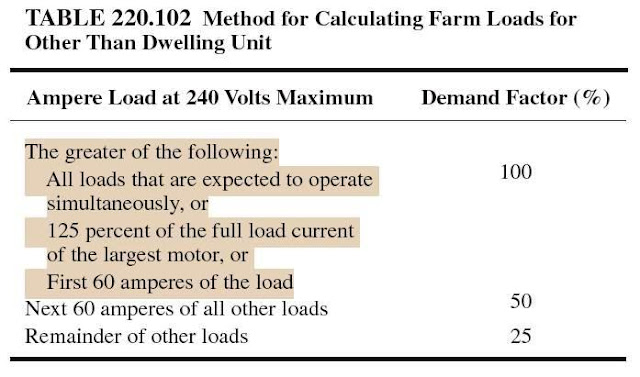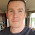# Electrical Load Calculations for Farms

Now, we arrive to Part V of Article 220, where the Electrical Load Calculations for Farms are discussed, you may note that farm load calculations are not part of the optional method load calculations because Farms usually have a dwelling unit and one or more additional buildings or structures that are not dwelling units.

Today, I will explain the load calculations for Farms as follows.

 Important!!! Farms usually have a dwelling unit and one or more additional buildings or structures (Other than dwelling units). So, Total Farm load will include two loads; Farm Dwelling Load and Farm Loads.

 Rule#1: Farms Electrical Load Calculations As per NEC Part V of Article 220, Farms Electrical Load Calculations will vary based on the existing case of the following: Case#1: If the Farm Dwelling load and Farm Loads are supplied by separate services Case#2: If the Farm Dwelling load and Farm Loads are supplied by one Common service.

Case#1: If the Farm Dwelling load and Farm Load are supplied by separate services

 Rule#2: Electrical Load Calculation of Farm Dwelling Load As per NEC section 220.102(A), If the Farm Dwelling load and Farm Load are supplied by separate services, calculate the Farm dwelling Load by either Standard NEC Method in (Part III of Article 220) or Optional NEC Method (Part IV of Article 220).

 Rule#3: Electrical Load Calculation of Farm Loads (Other Than Dwelling) As per NEC section 220.102(B), If the Farm Dwelling load and Farm Load are supplied by separate services, and Where a feeder or service supplies a farm building or other load that has two or more separate branch circuits, the load for feeders, service conductors and service equipment shall be calculated in accordance with demand factors not less than indicated in Table 220.102.Procedures for calculating Farm Loads (Other Than Dwelling) with separate service

Calculating Farm Load (Other Than Dwelling) as per Table 220.12 will be done in four steps as follows:

Step#1: compare the three following loads and select the largest:

1. All the loads that are expected to operate simultaneously, or
2. 125 percent of the full load current of the largest motor, or
3. First 60 amperes of the load.
Step#2: Multiply the next 60A of all other Loads by 50 percent.

Step#3: Multiply the remaining loads by 25 percent.

Step#4: the service or feeder Loads for Farm Load = Sum of all loads from steps#1,2 and 3.

Example#1:

A farm dwelling and farm building will be supplied by separate services. What is the minimum size service for the farm loads? The total ampere rating for all the farm loads (including simultaneously operated loads) will be 385 amperes (A). All the loads that are expected to operate simultaneously will have a total rating of 65A. The largest motor will be a 10 horsepower (hp), 240-volt (V), single-phase motor. The farm service will be supplied by a 120/240V, single-phase system.

Solution:

Calculate the service for this farm equipment in accordance with Table 220.102.

Step#1: compare the three following loads and select the largest:

a- All the loads that are expected to operate simultaneously will be 65A.

b- In accordance with Table 220.102, multiply the full-load current of the largest motor by 125 percent. The full-load current for the 10-hp motor (from Table 430.248) is 50A. After multiplying by 125 percent, the rating is = 50 A x 125% = 62.5 A

c- The third load is the first 60A of the total load.

After comparing the three, the largest is 65A. This load will be added to the calculation at 100 percent.

The remaining farm loads = 385 A – 65 A = 320 A

Step#2: Multiply the next 60A of all other Loads by 50 percent

Multiply the next 60A by 50 percent = 60 x 50% = 30 A

The remaining farm loads = 320 A – 60 A = 260 A.

Step#3: Multiply the remaining loads by 25 percent.

The remainder of all other loads = 260 x 25% = 65 A

Step#4: the service or feeder Loads for Farm Load = Sum of all loads from steps#1, 2 and 3
The total rating after applying the demand factors = 65 + 30 + 65 = 160 A

This rating is not a standard rating. In accordance with 240.6(A), the next standard ampere rating higher than 160 is 200.

So, the minimum size service for the farm loads in this example is 200 A.

Case#2: If the Farm Dwelling load and Farm Load are supplied by one Common service.

 Rule#4: Electrical Load Calculation of Farm Dwelling Load As per NEC section 220.102(A), If the Farm Dwelling load and Farm Load are supplied by separate services, calculate the Farm dwelling Load as per below Table and according to existing case.

 Table for identifying used Method for Electrical Load Calculation of Farm Dwelling Load IF Farm Dwelling Load has Electric Heat No Electric Heat IF Farm Load has electric grain-drying systems Standard NEC Method Standard or Optional NEC Method has No electric grain-drying systems Standard or Optional NEC Method Standard or Optional NEC Method

 Rule#5: Electrical Load Calculation of Farm Loads As per NEC section 220.103, If the Farm Dwelling load and Farm Load are supplied by one Common service, apply demand factor of Table 220.103 for calculating the total Farm load.

 Important!!! For Rule#5 above, the largest Motor Load must be multiplied by 125% before applying demand factors of table 220.103.

 Rule#6: Common Service Farm Load As per Note under Table 220.103, Common Service Farm Load = Total Farm Loads (calculated as per Rule#5 in above) + Farm Dwelling Load (calculated as per Rule#4 in above)

Example#2:

One service will supply both a farm dwelling and farm equipment. What is the minimum size service for the following loads? All the loads that are expected to operate simultaneously will have a total rating of 60 amperes (A). The largest motor will be a 10 horsepower (hp), 240-volt (V), single-phase motor. Another load will be 50A. The remaining loads will be 92A. The total load for the dwelling, calculated in accordance with Part III in Article 220, is 110A. A 120/240V, single-phase system will supply the farm service.

Solution:

Multiply the full-load current of the largest motor by 125 percent. The full-load current for the 10-hp motor (from Table 430.248) is 50 A. After multiplying by 125 percent, the rating is = 50 A x 125% = 62.5 A.

- The motor load is the largest load. This load will be added to the calculation at 100 percent as per table 220.103.

The motor load = 62.5 A X 100% = 62.5 A

- The next largest load (all the loads that are expected to operate simultaneously) is 60A.

The second largest load after applying the demand factor of table 220.103 = 60 A x 75% = 45 A

- The third largest load is 50A.

The third largest load after applying the demand factor of table 220.103 = 50 A x 65% = 32.5 A

- The remaining load, after applying the demand factor of table 220.103 = 92A x 50% = 46 A

The total load, after applying the demand factors of table 220.103 = 62.5 + 45 + 32.5 + 46 = 186 A

As specified in the note under Table 220.103, add the total farm loads (after demand factors) to the farm dwelling unit load.

In this example, the farm dwelling unit was calculated in accordance with Part III in Article 220 and the load is 110A.
The minimum ampere rating for the dwelling unit and farm equipment = 186 A+ 110 A= 296 A
This minimum rating is not a standard rating in accordance with 240.6(A). The next standard ampere rating higher than 296 is 300.

So, the minimum size service for the farm loads and dwelling unit load in this example is 300 A.

Example#3:

What is the minimum size service for a farm that will have the dwelling unit and four barns supplied by the one service? The total load for the dwelling, calculated in accordance with Part III in Article 220, is 147A. The barn loads are as follows: barn 1 = 103A; barn 2 = 84A; barn 3 = 72A; and barn 4 = 62A. A 120/240V, single-phase system will supply the farm service.

Solution:

Calculate the barn loads separately from the dwelling unit load then multiply the barn loads by the demand factor percentages in Table 220.103.

- The largest load is 103A

The largest load after applying the demand factor of table 220.103 = 103 A x 100% = 103 A

- The second largest load is 84A

The second largest load after applying the demand factor of table 220.103 = 84 A x 75% = 63 A

- The third largest load is 72 A

The third largest load after applying the demand factor of table 220.103 = 72 A x 65% = 46.8 A

- The fourth largest load is 62A

The remaining load, after applying the demand factor of table 220.103 = 62A x 50% = 31 A
The total load after applying the demand factors = 103 + 63 + 46.8 + 31 = 243.8 A

The minimum ampere rating for the dwelling unit and the four barns = 243.8 + 147 = 390.8 A = 391 A

This minimum rating is not a standard rating in accordance with 240.6(A). The next standard ampere rating higher than 391 is 400.

So, the minimum size service for the farm loads in this example is 400 A.

In the next article, I will explain load calculations for Feeder or Service Neutral Load and Overcurrent Protection Calculations. Please, keep following.

1.Hello Eng. Ali,

The information provided is splendid which cannot be found anywhere across the web. It's an immense contribution of knowledge.
Moving, one doubt is "when to opt for Standard calculation method or Optional calculation method?".
For an instant, if the feeder current is beyond 100 A, can Optional calculation method be adopted by default?
Further, procedure for Optional calculation is quiet lengthy when compared to Standard calculation in some cases. On top of that there are too many conditions to be satisfied before opting Optional calculation method.
Thereby, it's a request to pls clear this queries.

2.What if the farm service is 480V? This exceeds the stipulation of 240V maximum in table 220.102. Does this section not apply to voltages over 240V?